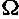### FORCED CONVECTION 1

#### TRANSIENT HEAT TRANSFER IN BOUNDARY-LAYER FLOWS

I. POP
Faculty of Mathematics, University of Cluj,
R-3400 Cluj, CP 253, Romania

The aim of this lecture is to review some of the existing results for the transient heat transfer in boundary-layer forced convection flows. The results outlined refer only to the transient heat transfer in a boundary-layer over a flat plate, past a wedge, and near a plane stagnation point, which are subject to a step change in wall temperature (impulsively heated walls). The governing equations, along with their important simplifications, are presented to indicate the dimensionless parameters that arise and the basic nature of the transient process. Theoretical expressions for the rate of heat transfer are presented in the form of graphs. In addition, a comprehensive list of papers is included, making the review paper useful for the future researcher in heat transfer.

#### CONCEPTS FOR UNSTEADY CONVECTIVE HEAT TRANSFER USING DEVICES WITHOUT MOVING PARTS

H. Herwig, I. Friedrich
Technische Thermodynamik, TU Chemnitz-Zwickau
Chemnitz, Germany

Three different concepts of unsteady heat and mass transfer based on unsteadily impinging jets are proposed. The physics of unsteady heat transfer is discussed with special emphasize on its heat transfer augmentation potential. A common feature of all three devices (processing jet, flip-flop jet and Karman vortex jet) is the absence of moving parts and external energy sources. Experimental results are shown and discussed for the processing jet arrangement.

#### THEORETICAL MODELISATION IN TRANSIENT CONVECTIVE HEAT TRANSFER FOR A LAMINAR BOUNDARY LAYER FLOW

M. LACHI, G. POLIDORI, N. CHITOU and J. PADET
Laboratoire de Thermomécanique, Faculté des Sciences
Moulin de la Housse - B.P. 1039, 51687 REIMS FRANCE

Based on the energy equation Formulation, a numerical simulation for characterizing the transient heat exchange between a steady laminar boundary layer and a flat sample is established. The unsteady behaviour is developed after generation of an impulsive heat flux step on the upper face of the plate. Two cases are considered according as the plate has a finite thickness or without thickness. The time and space evolution of both the surface temperature and the heat exchange coefficient is evidenced. The results are compared to the steady solution deduced from the KARMAN-POLHAUSEN method.

#### ANALYSIS OF TRANSIENT PERIODIC LAMINAR INTERNAL FORCED CONVECTION VIA ASYMPTOTIC SOLUTION OF COMPLEX EIGENVALUE PROBLEMS

Mazhar Ünsal
Department of Mechanical Engineering
University of Gaziantep, 27310 Gaziantep, Turkey

Laminar forced convection inside parallel-plate channels with periodic variation of inlet temperature is analysed. The Method of Matched Asymptotic Expansions (MMAE) is utilized to obtain a closed form asymptotic solution of the complex eigenvalue problem. The asymptotic solution presented is accurate for all eigenvalues when the dimensionless frequency of oscillation at inlet is small (<1). The asymptotic solution presented is inaccurate for small eigenvalues when the dimensionless frequency of oscillation at inlet is large (>1) but yields accurate predictions for large eigenvalues.

#### EXPERIMENTAL AND THEORETICAL ANALYSES OF UNSTEADY TURBULENT FORCED CONVECTION WITH TIMEWISE VARIATION OF INLET TEMPERATURE

M. Arýk1, C.A.C. Santos*2, and S. Kakaç3

Department of Mechanical Engineering
University of Miami
Coral Gables, FL 33124 - USA

*Department of Mechanical Technology
Joao Pessoa - PB 58059-900 - Brazil

Unsteady turbulent forced convection heat transfer in the thermally developing region of a parallel-plate channel with timewise varying inlet temperature is studied experimentally and theoretically. An experimental set-up was built and used in order to validate the employed mathematical modeling. For a wide range of Reynolds numbers and inlet frequencies are chosen in order to study the impact of inlet frequency on decay index. The theoretical solution of the problem consists of analytical and numerical parts. The analytical part is obtained through extending the generalized integral transform technique. The numerical part is employed after the analytical part is completed and a powerful method, Sing-Count, is used in order to solve the eigenvalue problem. Results obtained from both experimental and theoretical analyses have been presented in graphical and tabular forms. Analytical solutions are verified the experimental findings that is more close to the real world applications. Satisfactory agreement is obtained between theoretically and experimentally determined heat transfer characteristics for different axial positions along the channel.

1Graduate Student, Department of Mechanical Engineering, Research Assistant in the Department of Mechanical Engineering at Celal Bayar University, Manisa, Turkey
2Professor, Department of Mechanical Engineering
3Professor and Chairman, Department of Mechanical Engineering

#### NUMERICAL AND EXPERIMENTAL INVESTIGATION OF TRANSIENT LAMINAR FORCED CONVECTION IN A RECTANGULAR DUCT

Nedim SÖZBÝR+, Ünal UYSAL+, H. Ýbrahim SARAÇ++, Ýsmail ÇALLI+ and Sadýk KAKAÇ\$
+Department of Mechanical Engineering, Sakarya University, Sakarya,
++Department of Mechanical Engineering, Kocaeli University, Kocaeli,
\$Department of Mechanical Engineering, University of Miami, Coral Gables, FL, 33124, USA

This paper focuses on a numerical investigation and experimental study of transient laminar forced convection in a rectangular duct due to a sinusoidal heat input at the inlet with hydrodynamically developed and thermally developing air flow. The experiments were conducted over with range of Reynolds number (1120 =< Re =< 2220) and inlet frequencies (0.02Hz =< ß =< 0.24Hz) of the periodic heat input. The transient heat transfer problem is solved in the thermal entrance region of the duct. Numerical as well as experimental results are reported. A second order accurate explicit finite difference scheme is used in the numerical solution to the energy equation. Numerical results are obtained with the fully developed parabolic velocity profile under the boundary condition of the fifth kind which was verified by the experiments. Temperature variations along the centerline of the rectangular duct are observed to be thermal oscillations with the same frequency as the inlet periodic heat input and amplitudes that decayed exponentially with distance along the duct. The results are compared with those from experiments. Satisfactory agreement between the numerical and experimental results are obtained.